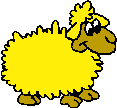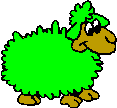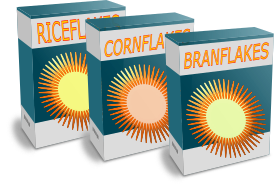# Exam-Style Questions on Ratio

## Problems on Ratio adapted from questions set in previous Mathematics exams.

### 1.

GCSE Higher

Sak needs 40 g of sugar to make 12 cakes.

He also needs four times as much flour as sugar and twice as much butter as sugar.

Sak is going to make 36 cakes.

(a) Work out the amount of butter he needs.

Sak has to buy all the flour he needs to make the cakes and he buys it in 500 g packs.

(b) How many packs of flour does Sak need to buy?

### 2.

GCSE Higher

If A is 150% more than B, what is the ratio A:B?

(a) $$1 : 150$$

(b) $$1 : 1.5$$

(c) $$2 : 3$$

(d) $$2 : 5$$

(e) $$5 : 2$$

### 3.

GCSE Higher

At a concert, the number of men, women and children can be expressed with the following ratio:

$$6 : 11 : 4$$

There were 6525 more women than men. How many children were at the concert?

### 4.

GCSE Higher

Mrs Painter makes a large amount of orange paint by mixing red and yellow paint in the ratio 3 : 4.

• Red paint costs £70 for 14 litres.
• Yellow paint costs £49 for 14 litres.Mrs Painter sells her orange paint in 14-litre tins for £62 per tin.

Calculate how much profit she makes for each tin she sells.

### 5.

GCSE Higher

Toystore sell model sheep in four different colours. In their newest warehouse there are currently 1760 sheep in stock. Three eighths of the sheep are yellow, 15% of the sheep are blue and the ratio of green to red sheep is 5:6.Work out the number of sheep of each colour in the Toystore warehouse.

### 6.

IGCSE Extended

In January, a supermarket sold 12 624 boxes of breakfast cereal.

(a) The cost of a box of cereal was £2.15 . Calculate the amount received from the sale of the 12 624 boxes. Give your answer correct to the nearest hundred pounds.

(b) The number of boxes sold in February was 13% more than the number sold in January. Calculate the number of boxes sold in February.(c) There were 3 different types of cereal. The number of boxes sold of each type every month was in the ratio Riceflakes : Cornflakes : Branflakes = 4 : 5 : 3. The total number of boxes sold was 12 624. Calculate the number of boxes of Branflakes sold.

(d) How may more boxes of Cornflakes than Riceflakes were sold in February?

(e) $$\frac{5}{6}$$ of the 12 624 bottles sold in January are included in a special promotion. Calculate the number of boxes that are included in that promotion.

### 7.

GCSE Higher

Promise is going to make some chocolate pyramids to sell.

The ingredients are chocolate, honey butter and cornflakes. The ingredients are mixed in the ratio $$5:2:3$$ respectively.

(a) How much of each ingredient will she need to make 800g of the mixture?

• A bar of chocolate weighs 200g and costs £2.80.
• A jar of honey butter contains 320g and costs £1.90.
• A packet of cornflakes contains 300 g and costs £2.40.

Promise makes 3.2 kg of the mixture, from which she can make 80 chocolate pyramids.

She charges 70p for each pyramid and sells all 80 of them.

(b) How much profit does she make?

### 8.

GCSE Higher

Pink paint is made by mixing red paint and white paint in the ratio 7 : 4

Mr Finklestein has 40 litres of red paint and 20 litres of white paint.

What is the maximum amount of pink paint he can make?### 9.

GCSE Higher

£580 is shared between Alice, Bally, Chris and Dean.

The ratio of the amount Alice gets to the amount Bally gets is 4 : 5

Chris and Dean each get 2.5 times the amount Alice gets.

Work out the amount of money that Bally gets.

### 10.

GCSE Higher

A shop sells mini packs of fruit gums of different sizes

• Orange fruit gums are sold in packs of 3.
• Strawberry fruit gums are sold in packs of 4.
• Banana fruit gums are sold in packs of 8.

On one busy day the ratio of packs of orange, strawberry and banana fruit gums sold was 4:5:6.

If total of 400 fruit gums were sold work out the number of packs of banana fruit gums sold

### 11.

GCSE Higher

An Airbus A380 plane is used by Tran Tours to fly between Dubai and London and holds a maximum of 740 passengers. The seating has been configured so that 15% of the seats are business class and the rest are economy class.

On one full flight, the ratio of males to females was 3:2. One eigth of the females were in business class.

How many males were in economy class?### 12.

GCSE Higher

The ratio a : b is equivalent to the ratio 7 : 3 and 8b = 5c

Find the ratio a : c in its simplest form.

### 13.

GCSE Higher

At a summer camp the campers can choose one of three programmes: camp craft, water sports or hiking.

During the first week of camp the campers chose the programmes in the ratio 7 : 5 : 8

During the second week of camp the same number of campers chose the programmes in the ratio 7 : 6 : 4

Show that there were more campers on the camp craft programme in the second week than the first.

### 14.

GCSE Higher

Given that:

$$x^2 : (5x + 3) = 1 : 3$$

find the possible values of $$x$$.

### 15.

GCSE Higher

There are some round counters and some square counters in a pot. The counters are either blue or green.

• The ratio of the number of round counters to square counters is 5:6
• The ratio of the number of blue counters to green counters is 2:7

(a) What is the least possible number of counters in the pot?

(b) If all the round counters are green work out the least possible number of square green counters that are in the pot.

### 16.

GCSE Higher

The points A, B C and D lie in order on a straight line.

$$AB:BD = 1:4$$ $$AC:CD = 9:11$$

Using the information above calculate the three part ratio:

$$AB:BC:CD$$

### 17.

GCSE Higher

The prices of two watches are in the ratio $$a:b$$.

When the prices are both increased by £10, the ratio becomes $$5 : 7$$.

When the prices are both reduced by £10, the ratio becomes $$1 : 3$$.

Express the ratio $$a:b$$ in its lowest terms.

### 18.

GCSE Higher

The surface gravity, $$g$$, of a planet is the gravitational acceleration experienced at its surface. The following formula can be used to find how a planet's gravity compares to Earth's.

$$g=\frac{6.67 \times 10^{-11} \times m}{r^2}$$

Where $$m$$ is the mass of the planet and $$r$$ is the radius.

Find the ratio of the surface gravity on Jupiter and the surface gravity Mars. Give your answer in the form $$n:1$$.

 Mass (kg) Radius (m) Jupiter 1.90 × 1027 6.68 × 107 Mars 6.39 × 1023 3.39 × 106

If you would like space on the right of the question to write out the solution try this Thinning Feature. It will collapse the text into the left half of your screen but large diagrams will remain unchanged.

The exam-style questions appearing on this site are based on those set in previous examinations (or sample assessment papers for future examinations) by the major examination boards. The wording, diagrams and figures used in these questions have been changed from the originals so that students can have fresh, relevant problem solving practice even if they have previously worked through the related exam paper.

The solutions to the questions on this website are only available to those who have a Transum Subscription.

Exam-Style Questions Main Page

Search for exam-style questions containing a particular word or phrase:

To search the entire Transum website use the search box in the grey area below.